# Constant of Proportionality Lesson Plan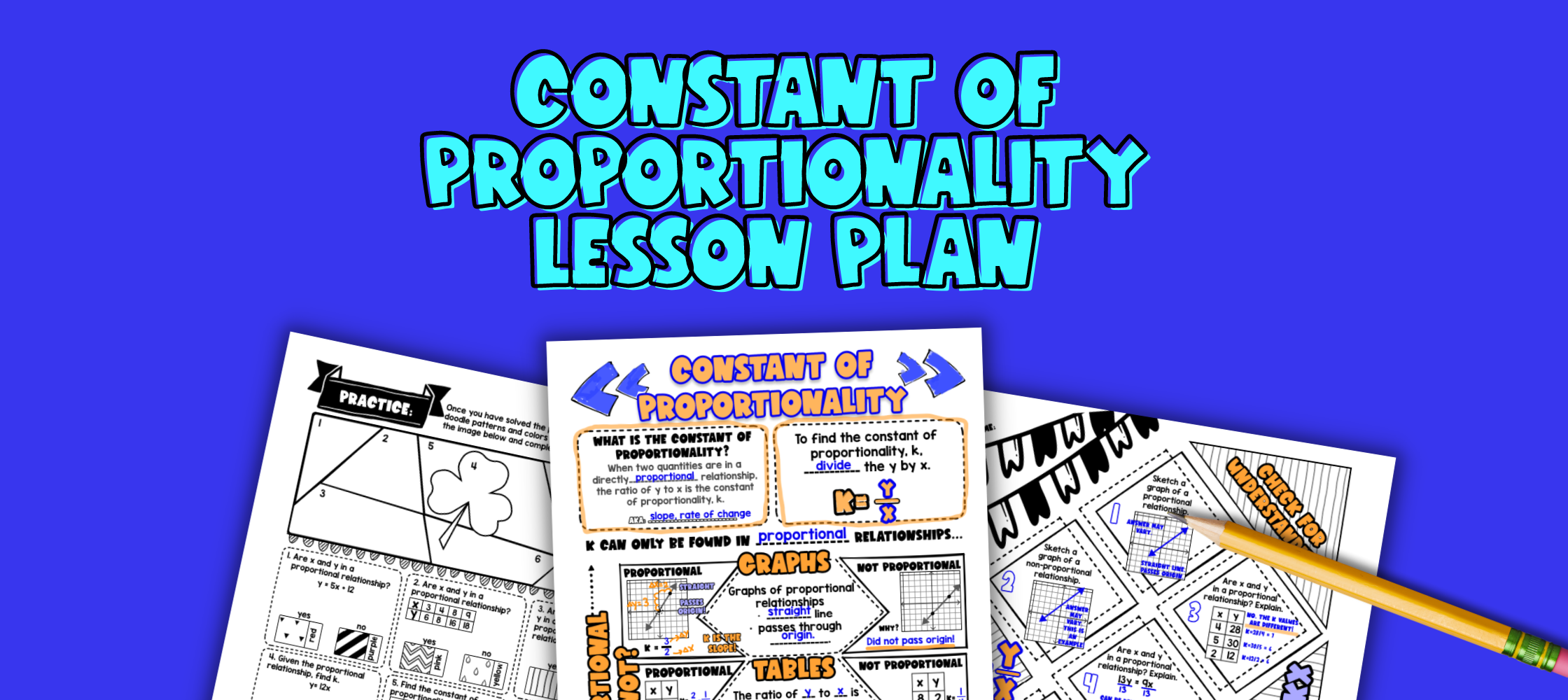Pin This

## Overview

Ever wonder how to teach constant of proportionality to your students? Looking for ideas to make it relevant to your students' life?

Use this artistic, real-life lesson plan to teach your students about the constant of proportionality and how to find k from graphs, equations, and tables.

Students will learn how constant of proportionality explains why their cell phone charger gets so hot, and why different devices take different amounts of time to charge. This lesson plan introduces the topic with guided notes (like sketch notes), practices the topic with a doodle & color by number worksheet and a maze activity, and wraps up with the real life application of constant of proportionality for cell phone chargers.

## Get the Lesson Materials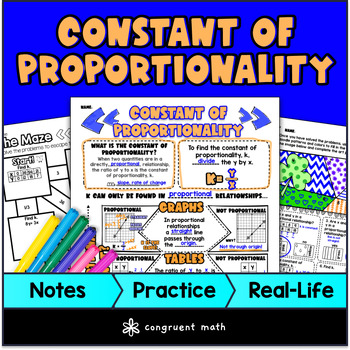\$4.25

## Learning Objectives

After this lesson, students will be able to:

• Identify proportional relationships in tables, graphs, and equations.

• Determine the constant of proportionality (k) for a given proportional relationship.

• Apply the concept of constant of proportionality to real-life scenarios, such as cell phone chargers and other electronic devices that require charging.

## Prerequisites

Before this lesson, students should be familiar with:

• Ratios and proportions

• Graphing on a coordinate plane

• Equivalent ratios and their relationship to proportional relationships

## Key Vocabulary

• Proportional relationship

• Constant of proportionality

• Unit rate

• Equivalent ratios

• Coordinate plane

• Graph

## Procedure

### Introduction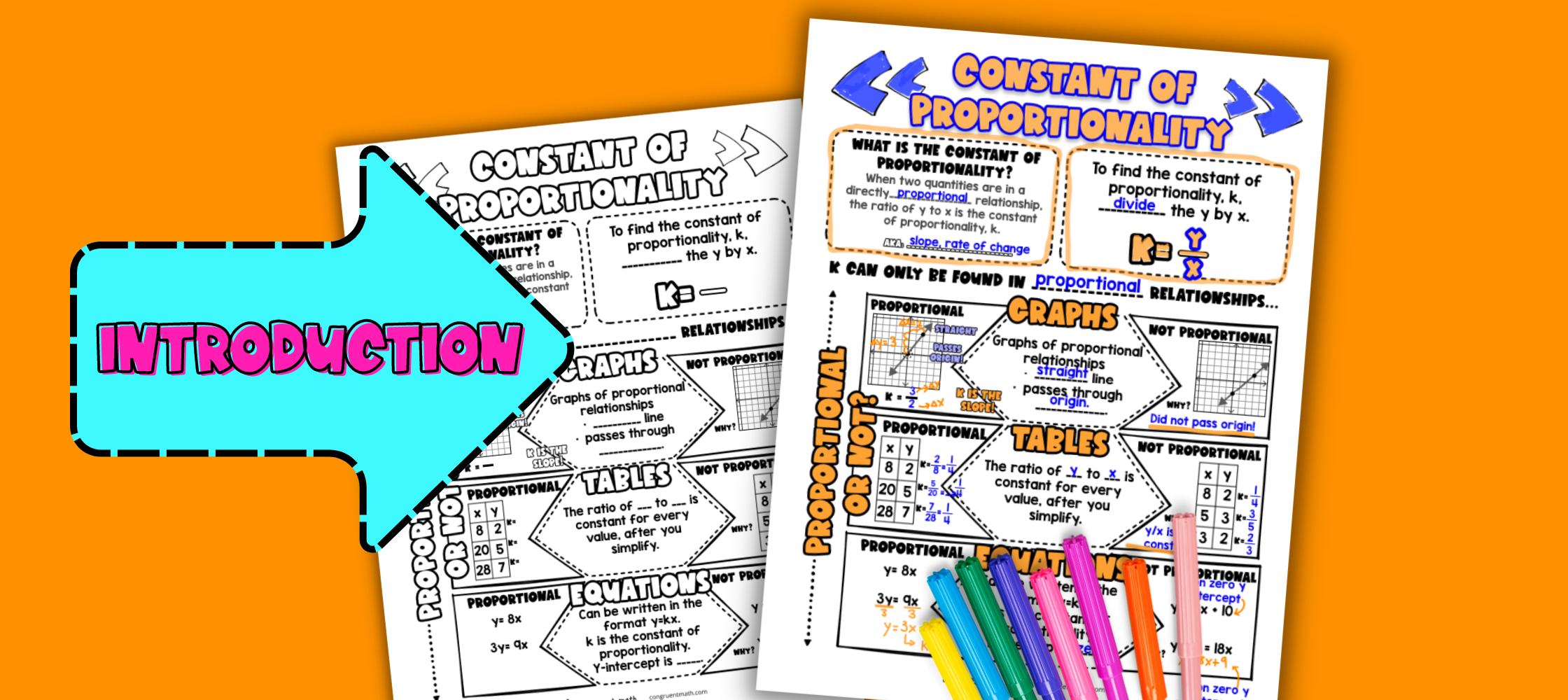Pin This
• As a hook, ask students why your cell phone charger gets so hot, or how different devices plugged into the same charger take different amounts of time to charge. Refer to the last page of the guided notes as well as the FAQs below for ideas.

• Use the guided notes to introduce the concept of proportional relationships and the constant of proportionality. Refer to the FAQ below for a walk through on this, as well as ideas on how to respond to common student questions.

• Walk through how to determine whether something is a proportional relationship and what the constant of proportionality is in tables, graphs, and equations.

### Check for Understanding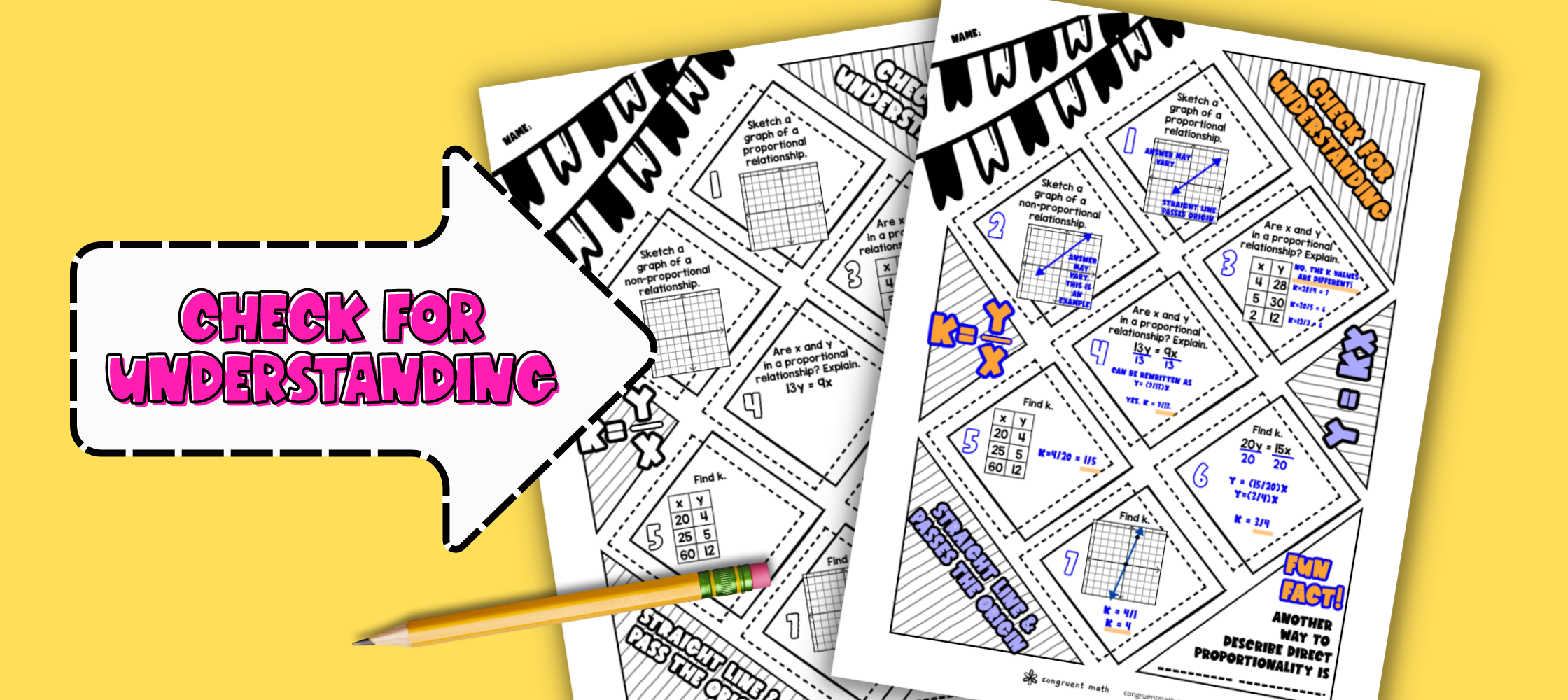Pin This
• Have students work through the problems on the check for understanding page, either collaboratively or independently.

• Walk around and spot-check student answers on the check for understanding activity.

• Based on student responses, reteach concepts that students need extra help with. If your class has a wide range of proficiency levels, you can pull out students for reteaching, and have more advanced students begin work on the practice exercises.

### Practice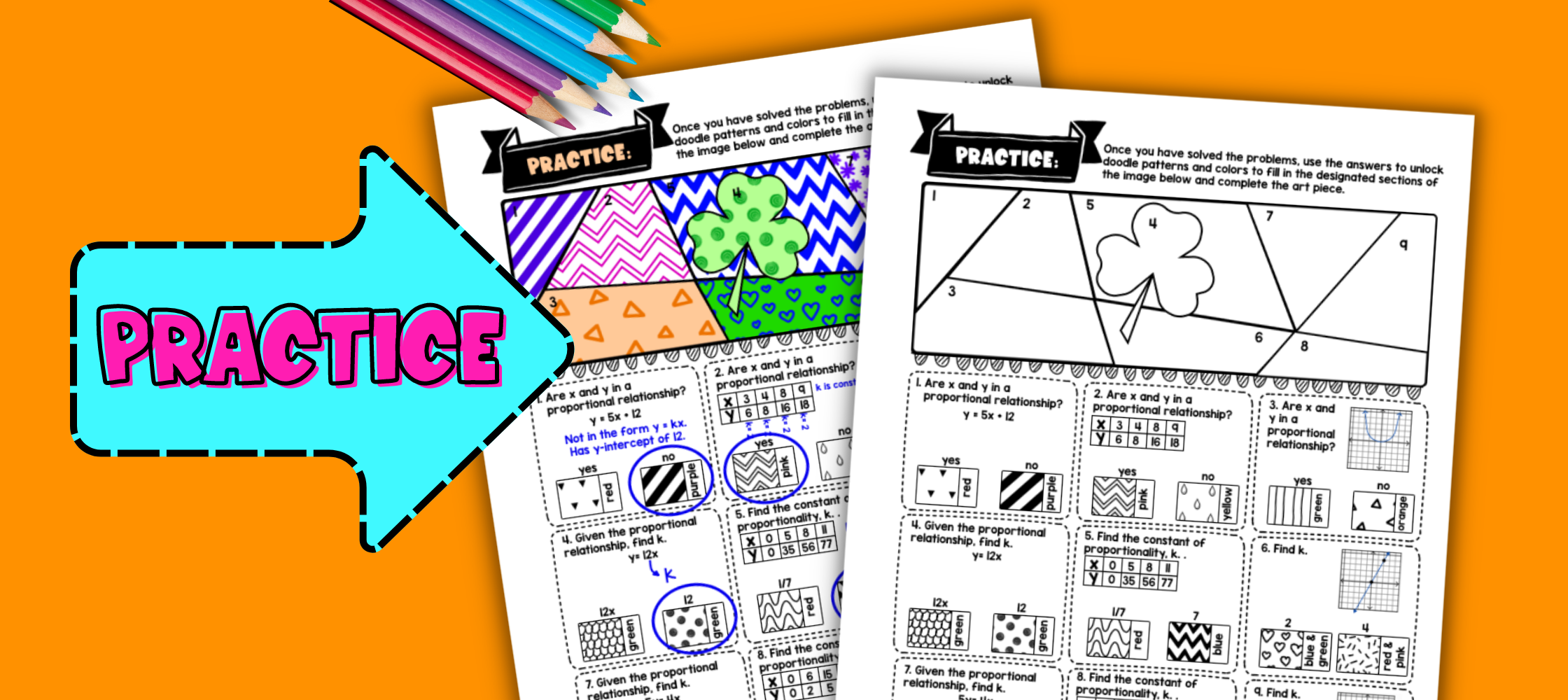Pin This
• Have students practice finding the constant of proportionality using the Doodle Math activity. Walk around to answer student questions.

• Fast finishers can dive into the maze activity for extra practice. You can assign it as homework for the remainder of the class.

### Real-Life Application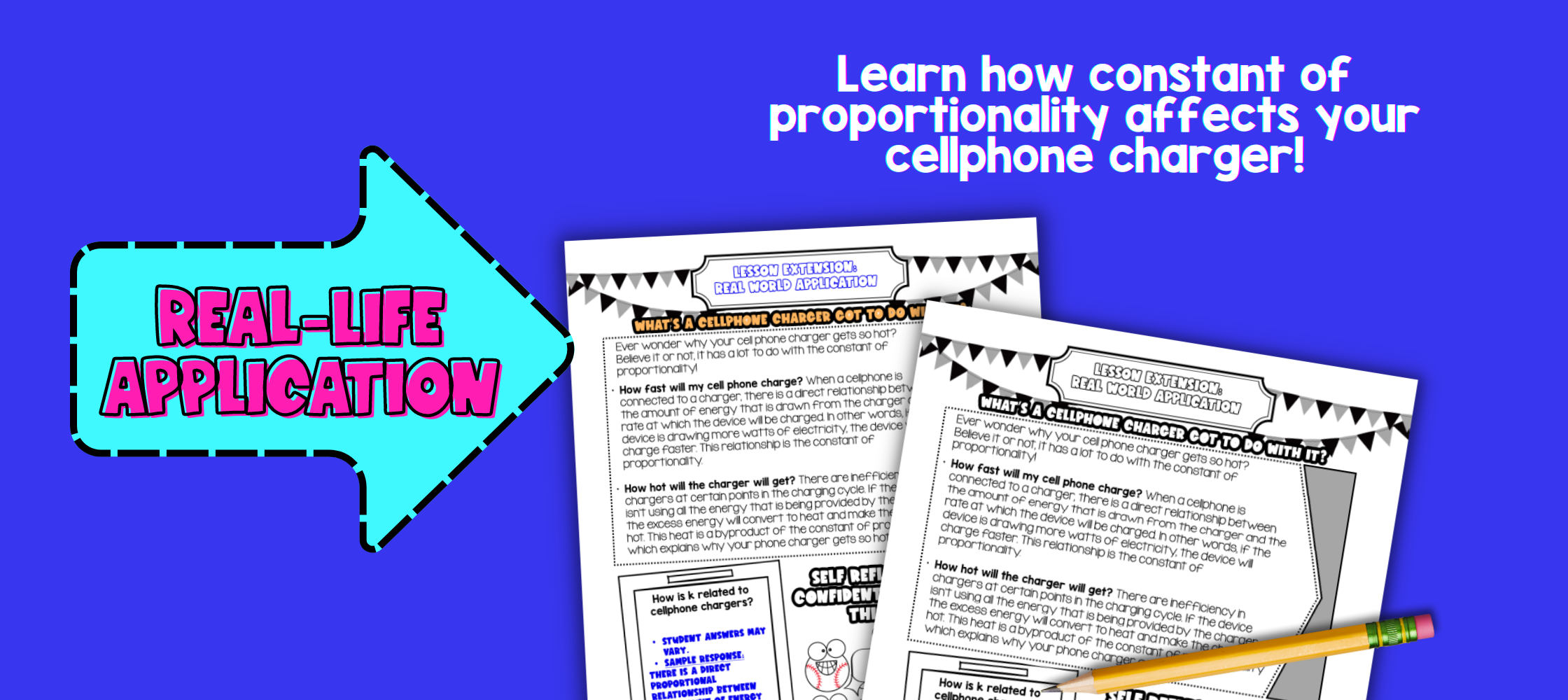Pin This
• Bring the class back together, and introduce the concept of how the constant of proportionality impacts how hot their cell phone charger gets and how fast it charges. Refer to the FAQ for more ideas on how to teach it, including a video!

## Extensions

If you’re looking for digital practice for constant of proportionality, try my Pixel Art activities in Google Sheets. Every answer is automatically checked, and correct answers unlock parts of a mystery picture. It’s incredibly fun, and a powerful tool for differentiation. There’s fall, winter, and spring versions perfect for additional practice.